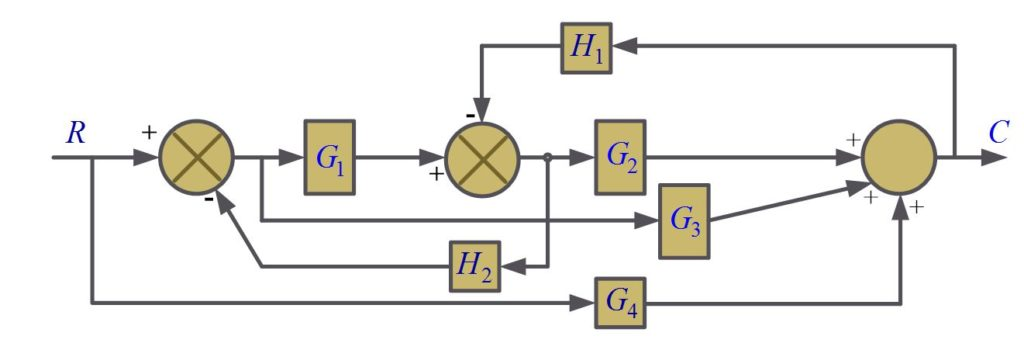Home / Control Systems / Signal Flow Graphs and Mason’s Gain Formula

Signal Flow Graphs and Mason’s Gain Formula

Want create site? Find Free WordPress Themes and plugins.

Signal Flow Graph

The application of mason’s gain formula to the signal flow graph corresponding to a given detailed block diagram is undoubtedly simplest operational procedure for obtaining the system transfer function. A signal flow graph is composed of various loops and one or more paths leading from an input to an output. Nodes representing system variables are interconnected by branches. Some important definitions and properties related to signals flow graph are given below:

Node:

Nodes represent system variables

Branch:

Branches are unidirectional paths that connect the nodes.

Input Node:

An input node has only outgoing branches.

Output Node:

And output node has only incoming branches.

Path:

A path is a continuous connection of branches with arrows in the same Directions

Loop:

A loop is a path that starts and ends on the same node with all other nodes in the loop touched only once.

Common Node:

A node that is contained in two or more loops.

Non- Touching loops:

Loops that have no common nodes.

Forward Path:

A forward path starts at an input node, ends at an output node, and touches no node more than once.

Gain:

Gains for paths and loops are defined as the products of branch gains for the paths or loops.

Mason gain’s formula is composed of three types of terms:

1. We identify all forward paths and designate the path gains as Pk for k=1,2,3,…
2. We form a parameter ∆ which denotes the interactions among the various loops.

∆=1-(sum of all single loops gains) + (sum of products of gains of all possible combinations of two non-touching loops)- (sum of products of gains of all possible combinations of three non-touching loops)+….

1. We form a parameter ∆k (for k=1,2,3,…) Which is a cofactor of kth forward path, obtained from ∆ by removing the loops that touch this path.

So mason’s gain formula for the overall gain is

$T=\frac{1}{\Delta }\sum\limits_{k=1,2,3,…}{{{P}_{k}}{{\Delta }_{k}}}$

Example of Signal Flow1. There are three forward paths from R to C and three loops, with gains

${{P}_{1}}={{G}_{1}}{{G}_{2}}$

${{P}_{2}}={{G}_{3}}$

${{P}_{3}}={{G}_{4}}$

${{L}_{1}}=-{{G}_{1}}{{H}_{2}}$

${{L}_{2}}=-{{G}_{2}}{{H}_{1}}$

${{L}_{3}}={{G}_{3}}{{H}_{1}}{{H}_{2}}$

1. All three loops touch, so ∆ is 1 minus sum of the loop gains

$\Delta =1-(-{{G}_{1}}{{H}_{2}}-{{G}_{2}}{{H}_{1}}+{{G}_{3}}{{H}_{1}}{{H}_{2}})$

$\Delta =1+{{G}_{1}}{{H}_{2}}+{{G}_{2}}{{H}_{1}}-{{G}_{3}}{{H}_{1}}{{H}_{2}}$

1. Special Case

If all forward paths and all loops touch each other, it may be seen that the cofactors are all unity.

In this example, the forward paths P1 and P2 touch all three loops so the corresponding cofactors ∆1 and ∆2 are unity. However, loop L1 does not touch path P3 so;

${{\Delta }_{3}}=1+{{G}_{1}}{{H}_{2}}$

Substituting values from above all three steps into the gain formula yields the closed loop transfer function.

$T=\frac{{{P}_{1}}{{\Delta }_{1}}+{{P}_{2}}{{\Delta }_{2}}+{{P}_{3}}{{\Delta }_{3}}}{\Delta }$

So,

$T=\frac{{{G}_{1}}{{G}_{2}}+{{G}_{3}}+{{G}_{4}}(1+{{G}_{1}}{{H}_{2}})}{1+{{G}_{1}}{{H}_{2}}+{{G}_{2}}{{H}_{1}}-{{G}_{3}}{{H}_{1}}{{H}_{2}}}$

Mason’s gain formula is preferable to determine transfer function either directly for simple systems or with the use of digital computers for large-scale systems having many feedback loops and forward paths.

Did you find apk for android? You can find new Free Android Games and apps.